HistoryMathematiciansAll formulasNum. approx.SoftwaresMisc. math.DigitsPoetryPapers/videosDelirium !Pi-DayImagesMusicLinksBibliography Boris Gourévitch The world of Pi - V2.57 modif. 13/04/2013HomeVersion historyGuestbookWho I amSome pictures (fr)AcknowledgementsLast modificationsContact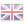Benoit Cloitre

##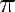andin a mirror

Benoit Cloitre, still very creative, is pursuing his chronicles about resemblance between famous constants, after those betweenet.

### 1 ForLetand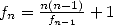then. Thus, if we write downthen we obtain an inverse Brounker-like continued fraction.

### 2 ForLetand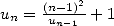then. The equivalent continued fraction is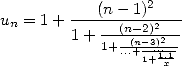### Proof for Pi

It is easy to see by induction thatand we recognise the Wallis product.

### Proof forLet. We easily see that for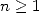,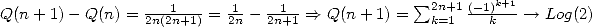.

### Checking under the software Pari-GP

For Pi

x=171679;u=x;for(n=2,100,u=n*(n-1)/u+1;if(n%2==0,print1((u-n-1)/(u-n)-prod(k=1,n/2,4*k^2/(4*k^2-1))*(1+1/n)*(x-1)/(x-2),",")))

,0,0,0,0,0,0,0,0,0,0,0,0,0,0,0,0,0,0,0,0,0,0,0,0,0,0,0,0,0,0,0,0,0,0,0,0,0,0,0,0,0,0,0,0,0,0,

For log(2)

f(n)=if(n<2,171679,(n-1)^2/f(n-1)+1);Q(n)=1/(2*n-f(2*n))-1/(171679-1);for(n=1,10,print1(Q(n+1)-sum(k=1,2*n+1,(-1)^(k+1)/k),","))

0,0,0,0,0,0,0,0,0,0,